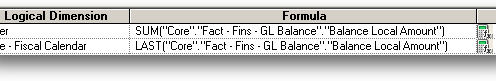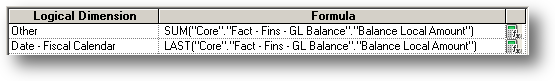# Aggregation Based on Dimensions in OBIEE

The Business Model in OBIEE allows for measures to be aggregated using a variety of different functions. For most scenarios this is sufficient. For example, if we are recording sales by month, then SUM(sales) will allow us to report on those amounts at a higher level such as year.
Consider the following sample dataset.

 SalesPerson Month Name Sales Amount Alice Jan 1000 Alice Feb 2000 Alice Mar 1500 Bob Jan 2000 Bob Feb 100 Bob Mar 5000

It would be trivial to return the total sales for each salesperson using a SUM function on the Sales Amount measure.

 SalesPerson Sales Amount Alice 4500 Bob 7100

However; what if we also had a “Year to Date” column, which was a running total of sales in the current year?

 SalesPerson Month Name Sales Amount Sales YTD Alice Jan 1000 1000 Alice Feb 2000 3000 Alice Mar 1500 4500 Bob Jan 2000 2000 Bob Feb 100 2100 Bob Mar 5000 7100

Clearly we cannot SUM this column, because the values for each month includes the previous months figures too. What we probably want (I say probably as different cases of course have different requirements) is to pick the latest amount for each salesperson. We could pick LAST as a rule, but then that wouldn’t SUM up the sales for Alice and Bob – i.e. we would get an answer of 7100 rather than (7100+4500).

What we need is Aggregation Based on Dimensions. This is a feature available in the properties of the logical column under the aggregation tab. Tick the “Based on Dimensions” checkbox.
This allows multiple aggregation rules to be applied to a column. So – for the example above – if I use the following rule…

 Dimension Formula Other SUM(Sales Amount) Month Name LAST(Sales Amount)

… this says “By default (Other), SUM the values, however for the Calendar Date dimension pick the LAST value”. It is easier to visualize what actually happens by considering the SQL code that the BI Server generates.

Assume I create an analytic which just displays the Sales YTD column. These are the steps that the BI Server goes through.

1) Generate a temporary view (as in a materialized WITH clause, not a database view object) of the data, grouped by the dimensions selected in the analytic, and the dimensions in the aggregation rule. The grouping will use the Other rule.

SELECT Month_Name, SUM(Sales_YTD) FROM my_table GROUP BY Month_Name;

 Month Name SUM(Sales_YTD) as C1 Jan 3000 Feb 5100 Mar 11600

2) Push the results of this into a query which applies the next aggregation rule, removing the dimension that is being aggregated over (Month Name) by grouping, using the aggregation function specified in the rule.

SELECT LAST(C1) OVER (ORDER BY Month_Key) FROM {Results of Step 1}

 LAST(C1)… as C2 11600 11600 11600

3) Finally, because the function LAST is an analytic function and therefore rows are not removed, the BI Server will collapse the result set into a single row (the rows all have the same value so any function such as MIN/MAX will suffice).

 MAX(C2) 11600

We get a value of 11600 which is what we expected. Obviously this is a trivial example, however it is found in the real world. For example the measure Fact – Fins – GL Balance.Balance Local Amount measure (amongst others) in BI Applications uses exactly this.This technique is not just used for Dates. Say we create a subject area based on Invoices/Lines in Oracle E-Business Suite. We need information from the lines however the invoice amount is stored against the invoice record. If our granularity is line level then we cannot SUM all amounts as we will get a result that is an order of magnitude too large based on the number of lines that invoice has! We therefore need to aggregate out the lines (using any function that will keep the value the same) before SUM’ing the amounts. A typical example of this would be:

 Dimension Formula Other SUM(Amount) Invoice Line MAX(Amount)

If you are unsure whether you need to use aggregation by dimensions then there are a couple of general cases which may indicate the need to do so.

1) Your measure column is a running total
2) You are aggregating a column that comes from a source which is at a higher level of granularity than the granularity of the fact. I.e. you get multiple rows with the same value repeated in the underlying table (in the example above, the invoice amount is repeated on every invoice line record).

The simple examples in this blog only use a two rules (including Others) however you can of course use an many as required. I may elaborate in the future with some more complex examples.

This site uses Akismet to reduce spam. Learn how your comment data is processed.